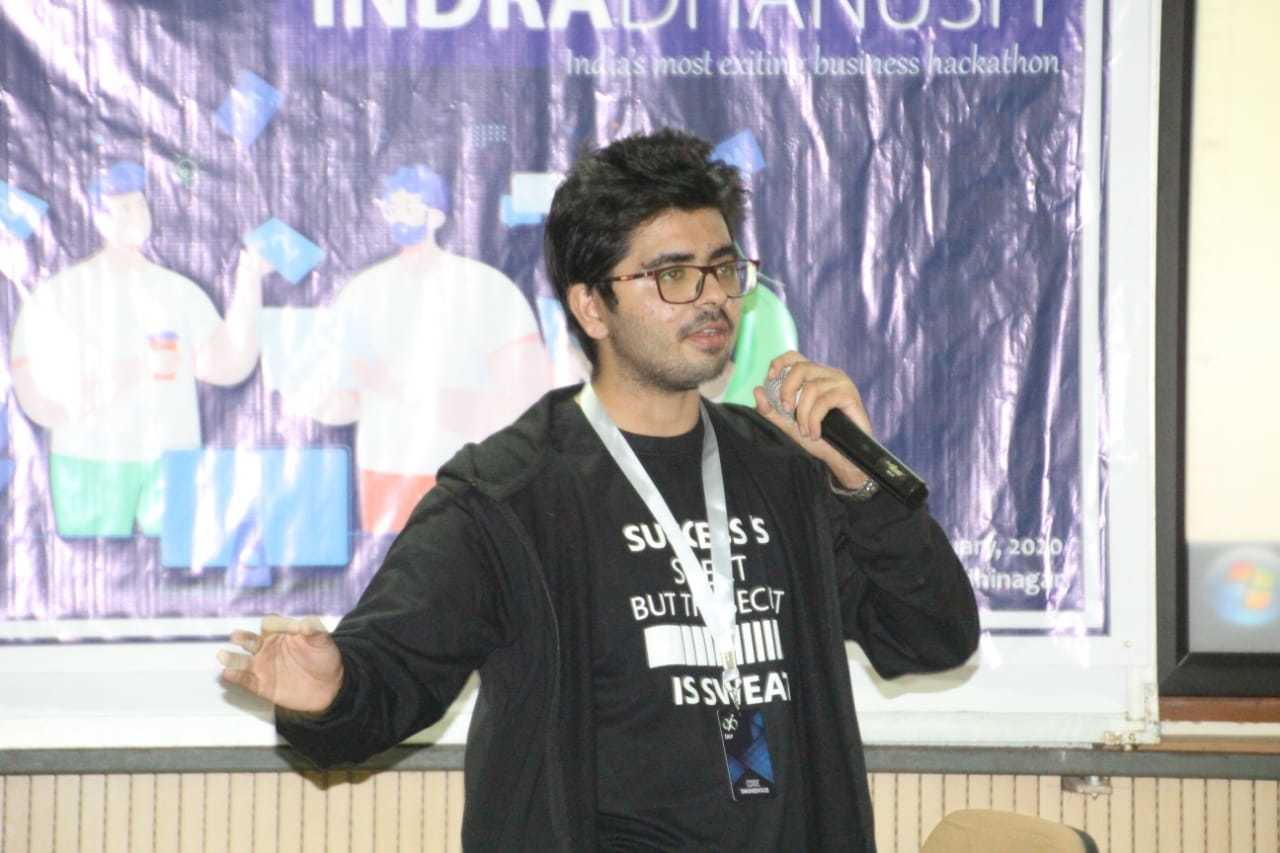# Count occurrences of a sub-string with one variable character

Given two strings a and b, and an integer k which is the index in b at which the character can be changed to any other character, the task is to check if b is a sub-string in a and print out how many times b occurs in a in total after replacing the b[k] with every possible lowercase character of English alphabet.

Examples:

Input: a = “geeks”, b = “ee”, k = 1
Output: 1
Replace b with ‘k’ and “ek” is a sub-string in “geeks”
“ee” is also a sub-string in “geeks”
Hence the total count is 2

Input: a = “dogdog”, b = “dop”, k = 2
Output: 2
Replace b with ‘g’, “dog” is a sub-string in “dogdog” which appears twice.

## Recommended: Please try your approach on {IDE} first, before moving on to the solution.

Approach: Make a list of all possible versions of the string b by iterating through all the lowercase letters and replacing the kth i.e. b[k] character in b with the current character.
Then count the number of occurrence of the new string b in the original string a and store it in a variable count. After all the lowercase characters are used, print the count.

Below is the implementation of the above approach:

 `# Python3 implementation of the approach ` `import` `string ` ` `  `# Function to return the count of occurrences ` `def` `countOccurrence(a, b, k):     ` ` `  `    ``# Generate all possible substrings to ` `    ``# be searched  ` `    ``x ``=` `[] ` `    ``for` `i ``in` `range``(``26``): ` `        ``x.append(b[``0``:k] ``+` `string.ascii_lowercase[i] ``+` `b[k ``+` `1``:]) ` ` `  `    ``# Now search every substring 'a' and ` `    ``# increment count    ` `    ``count ``=` `0` `    ``for` `var ``in` `x: ` `        ``if` `var ``in` `a: ` `            ``count ``+``=` `a.count(var) ` `             `  `    ``return` `count ` ` `  `# Driver code ` `a, b ``=` `"geeks"``, ``"ee"` `k ``=` `1` `print``(countOccurrence(a, b, k)) `

Output:

```2
```

Attention reader! Don’t stop learning now. Get hold of all the important DSA concepts with the DSA Self Paced Course at a student-friendly price and become industry ready.

My Personal Notes arrow_drop_upFront and Back End Web Developer Android Developer Python and C++ Coder Networking Geek

If you like GeeksforGeeks and would like to contribute, you can also write an article using contribute.geeksforgeeks.org or mail your article to contribute@geeksforgeeks.org. See your article appearing on the GeeksforGeeks main page and help other Geeks.

Please Improve this article if you find anything incorrect by clicking on the "Improve Article" button below.

Improved By : nidhi_biet